Anúncio

# MWA 10: 1.3 Setting Price

3 de Sep de 2019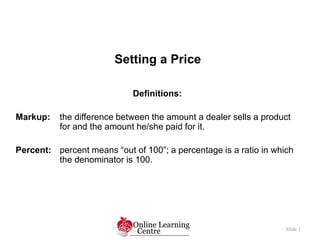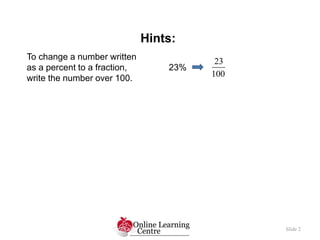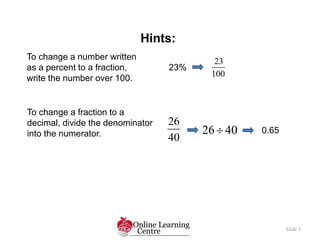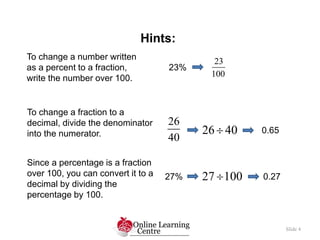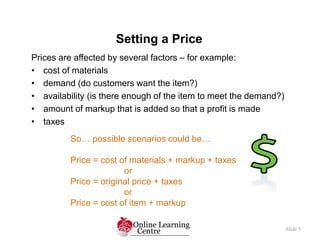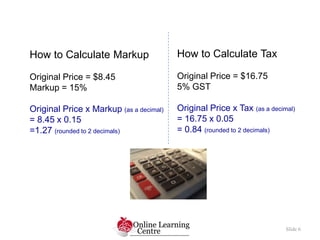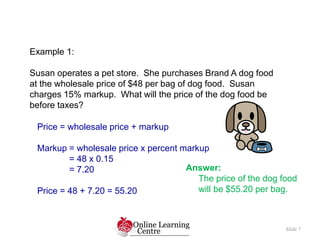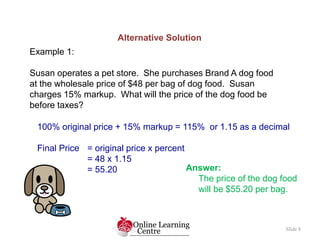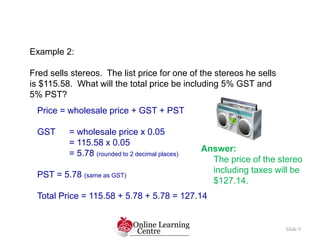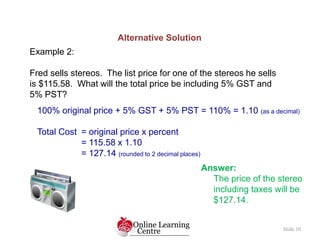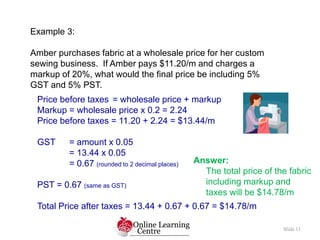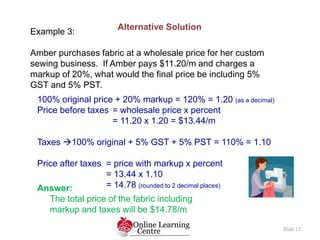1 de 12
Anúncio

### MWA 10: 1.3 Setting Price

1. Setting a Price Slide 1 Definitions: Markup: the difference between the amount a dealer sells a product for and the amount he/she paid for it. Percent: percent means “out of 100”; a percentage is a ratio in which the denominator is 100.
2. Hints: Slide 2 To change a number written as a percent to a fraction, write the number over 100. 23% 23 100
3. Hints: Slide 3 To change a number written as a percent to a fraction, write the number over 100. 23% 23 100 To change a fraction to a decimal, divide the denominator into the numerator. 26 40 26 40 0.65
4. Hints: Slide 4 To change a number written as a percent to a fraction, write the number over 100. 23% 23 100 To change a fraction to a decimal, divide the denominator into the numerator. 26 40 26 40 0.65 Since a percentage is a fraction over 100, you can convert it to a decimal by dividing the percentage by 100. 27% 27 100 0.27
5. Slide 5 Prices are affected by several factors – for example: • cost of materials • demand (do customers want the item?) • availability (is there enough of the item to meet the demand?) • amount of markup that is added so that a profit is made • taxes Setting a Price So… possible scenarios could be… Price = cost of materials + markup + taxes or Price = original price + taxes or Price = cost of item + markup
6. Slide 6 How to Calculate Markup Original Price = \$8.45 Markup = 15% Original Price x Markup (as a decimal) = 8.45 x 0.15 =1.27 (rounded to 2 decimals) How to Calculate Tax Original Price = \$16.75 5% GST Original Price x Tax (as a decimal) = 16.75 x 0.05 = 0.84 (rounded to 2 decimals)
7. Slide 7 Example 1: Susan operates a pet store. She purchases Brand A dog food at the wholesale price of \$48 per bag of dog food. Susan charges 15% markup. What will the price of the dog food be before taxes? Price = wholesale price + markup Markup = wholesale price x percent markup = 48 x 0.15 = 7.20 Price = 48 + 7.20 = 55.20 Answer: The price of the dog food will be \$55.20 per bag.
8. Slide 8 Example 1: Susan operates a pet store. She purchases Brand A dog food at the wholesale price of \$48 per bag of dog food. Susan charges 15% markup. What will the price of the dog food be before taxes? 100% original price + 15% markup = 115% or 1.15 as a decimal Final Price = original price x percent = 48 x 1.15 = 55.20 Alternative Solution Answer: The price of the dog food will be \$55.20 per bag.
9. Slide 9 Example 2: Fred sells stereos. The list price for one of the stereos he sells is \$115.58. What will the total price be including 5% GST and 5% PST? Price = wholesale price + GST + PST GST = wholesale price x 0.05 = 115.58 x 0.05 = 5.78 (rounded to 2 decimal places) PST = 5.78 (same as GST) Total Price = 115.58 + 5.78 + 5.78 = 127.14 Answer: The price of the stereo including taxes will be \$127.14.
10. Slide 10 Example 2: Fred sells stereos. The list price for one of the stereos he sells is \$115.58. What will the total price be including 5% GST and 5% PST? 100% original price + 5% GST + 5% PST = 110% = 1.10 (as a decimal) Total Cost = original price x percent = 115.58 x 1.10 = 127.14 (rounded to 2 decimal places) Alternative Solution Answer: The price of the stereo including taxes will be \$127.14.
11. Slide 11 Example 3: Amber purchases fabric at a wholesale price for her custom sewing business. If Amber pays \$11.20/m and charges a markup of 20%, what would the final price be including 5% GST and 5% PST. Price before taxes = wholesale price + markup Markup = wholesale price x 0.2 = 2.24 Price before taxes = 11.20 + 2.24 = \$13.44/m GST = amount x 0.05 = 13.44 x 0.05 = 0.67 (rounded to 2 decimal places) PST = 0.67 (same as GST) Total Price after taxes = 13.44 + 0.67 + 0.67 = \$14.78/m Answer: The total price of the fabric including markup and taxes will be \$14.78/m
12. Slide 12 Example 3: Amber purchases fabric at a wholesale price for her custom sewing business. If Amber pays \$11.20/m and charges a markup of 20%, what would the final price be including 5% GST and 5% PST. 100% original price + 20% markup = 120% = 1.20 (as a decimal) Price before taxes = wholesale price x percent = 11.20 x 1.20 = \$13.44/m Taxes 100% original + 5% GST + 5% PST = 110% = 1.10 Price after taxes = price with markup x percent = 13.44 x 1.10 = 14.78 (rounded to 2 decimal places) Alternative Solution Answer: The total price of the fabric including markup and taxes will be \$14.78/m
Anúncio